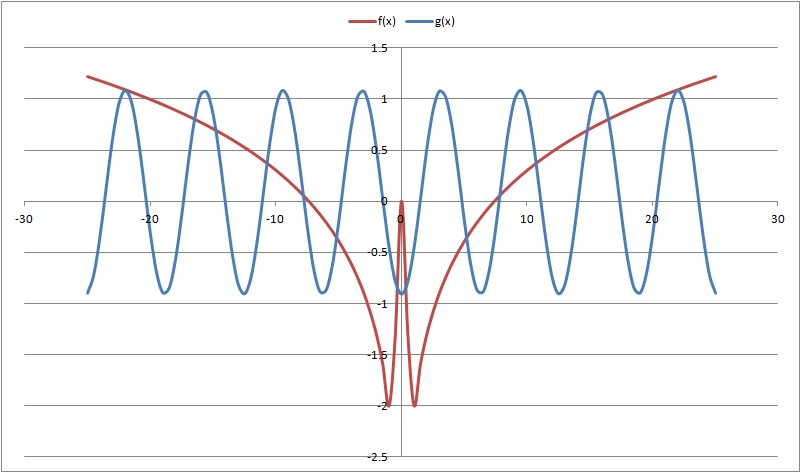# Complex sign. I mean sine!

Algebra Level 4$\large \frac{\pi}{A} - i \ln {\left(B+\sqrt{C}\right)}$

There is no real value for $\sin^{-1}(2)$ but it has a complex one. And it is of the form as described above where, $A$, $B$ and $C$ are positive integers. Evaluate $A+B+C$.

×